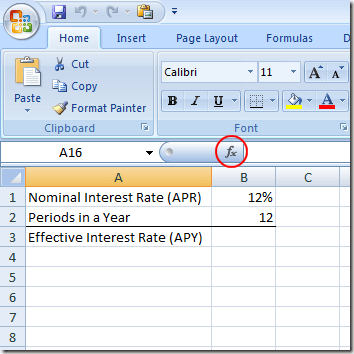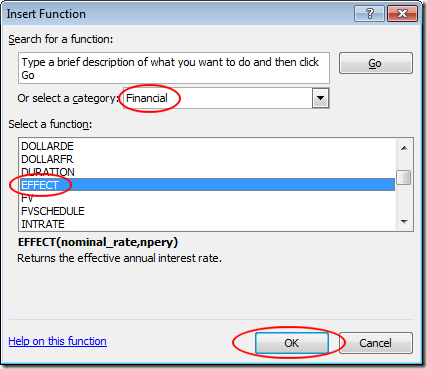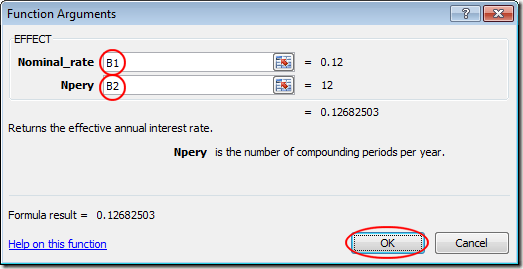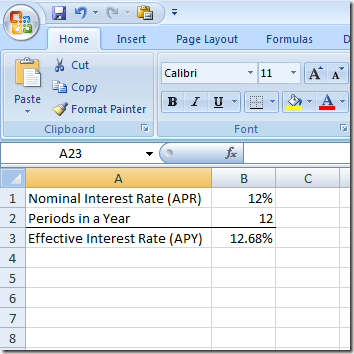Among Excel’s more popular formulas, the EFFECT formula is often used by financial professionals to figure out an effective interest rate from a nominal interest rate.

Also called annual percentage rate (APR) and annual percentage yield (APY), Excel makes it easy to calculate effective mortgage, car loan, and small business loan interest rates from the nominal rates often quoted by lending institutions.

## Effective vs. Nominal Interest Rates

Nominal interest rates are often quoted by lending institutions because they can make the cost of a loan appear lower than if the actual cost of the loan were quoted. This is because there are normally multiple payments and interest calculations made in a year.

Suppose you take out a loan that requires monthly payments. As a result, interest is calculated monthly as well. The nominal interest rate, also called annual percentage rate (APR), is simply the monthly interest rate (say 1% per month) multiplied by twelve (the number of periods in a year). This words out to a 12% interest rate.However, since interest is compounded monthly, the actual or effective interest rate is higher because interest in the current month compounds against interest in the previous month.

As it turns out, a 12% APR (nominal) interest loan has an effective (APY) interest rate of about 12.68%.

On a loan with a life of only one year, the difference between 12% and 12.68% is minimal. On a long-term loan such as a mortgage, the difference can be significant.

Read on to learn how to use Excel’s EFFECT formula to calculate an effective interest rate (APY) from a nominal interest rate (APR).

## Use Excel’s EFFECT Formula

Suppose you want to figure out the effective interest rate (APY) from a 12% nominal rate (APR) loan that has monthly compounding. You have set up your Excel worksheet to look like the one below.Notice that we have the nominal interest rate (APR) in cell B1 and the number of payment periods in cell B2.

To figure out the effective interest rate (APY), click on the cell at B3, click on the Insert Function button, and choose Financial from the drop down menu labeled Or Select a Category.

Locate and click on the function titled EFFECT and then click the OK button.This will open up the Functions Argument window. In the Nominal_rate box, type in B1 and in the Npery box, type in B2. Then, click the OK button.Notice that Excel places the figure 0.1268 in the B3 cell. If you prefer, you can change the format of the B3 cell to a percent.Note that now you can change the values in both B1 and B2 and Excel will calculate the effective interest rate (APY) in cell B3. For example, change the nominal interest rate (APR) in B1 to 6% and the effective interest rate (APY) in B3 changes to 6.17%.

Using the EFFECT function in Excel, you can figure out any effective interest rate given any nominal rate and the number of compounding periods in a year.